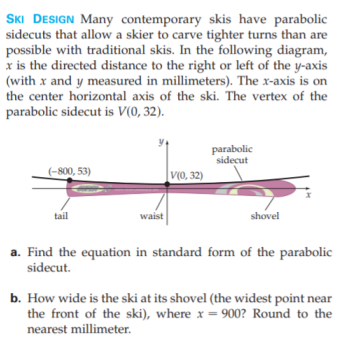### Still have math questions?

Algebra
QuestionSKI DESIGN Many contemporary skis have parabolic sidecuts that allow a skier to carve tighter turns than are possible with traditional skis. In the following diagram,

$$x$$ is the directed distance to the right or left of the $$y$$ -axis (with $$x$$ and $$y$$ measured in millimeters). The $$x$$ -axis is on the center horizontal axis of the ski. The vertex of the parabolic sidecut is $$V ( 0,32 )$$ . a. Find the equation in standard form of the parabolic sidecut. b. How wide is the ski at its shovel (the widest point near the front of the ski), where $$x = 900$$ ? Round to the nearest millimeter.

$$\text { a. } 4 ( \frac { 800 } { 84 } ) ^ { 2 } ( y - 32 ) = x ^ { 2 } \quad \text { b. } 117 \text { millimeters }$$